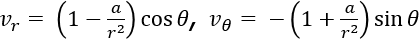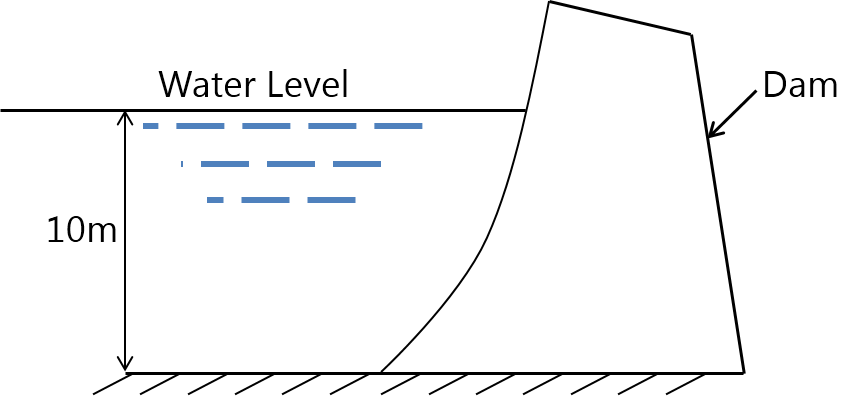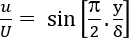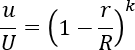MU Mechanical Engineering (Semester 4)
Fluid Mechanics
May 2013
Total marks: --
Total time: --
INSTRUCTIONS
(1) Assume appropriate data and state your reasons
(2) Marks are given to the right of every question
(3) Draw neat diagrams wherever necessary

1 (a) How fluids are classified? Represent different types of fluids on shear stress vs velocity gradient plot.
5 M
1 (b) A flow field is given by,i) Show that it represents a physically possible flow.
ii) Determine whether the flow is rotational or irrotational.
5 M
1 (c) Define displacement thickness in boundary layer theory and derive its relation in terms of dimensionless velocity profile, u/U,where u is the velocity at a perpendicular distance y from the surface of the plate and U be the free stream velocity.
5 M
1 (d) What is meant by grid skewness, grid independence in CFD?
5 M

2 (a) Find the magnitude and direction of the resultant water pressure acting on a curved face of a dam which is shaped according to the relation
y = x2/9 as shown in the figure. The height of the water retained by the dam is 10m. Consider the width of the dam as unity.8 M
2 (b) Using the Reynold's transport theorem and selecting appropriate control volume find the force exerted by the water on bend in magnitude and direction. The following data is supplied:
Pipe bend measures 30 cm at the entrance and 20 cm diameter at exit. Pipe bend angle 120° (Angle measured in anticlockwise direction from initial to final direction of flow with water from left hand side). Discharge = 0.25m3∕s, volume of the bend is 0.10m3 and pressure at the entrance = 60KPa gauge. The exit is 2m above the entrance section.
12 M

3 (a) A two dimensional flow is described in the Langrangian system as
x=x0e-kt + y0(1-e-2kt) and
y = y0ekt.
Find:
(i) The equation of the particle in the flow field
(ii) The velocity components in Eulerian system
10 M
3 (b) For the velocity profile for laminar boundary flowwhere u is the velocity at the distance y from the surface of the plate and U is the free stream velocity at the boundary layer thickness ?. Obtain an expression for boundary layer thickness and shear stress in terms of Reynolds number.
10 M

4 (a) Consider a two dimensional viscous incompressible flow of a Newtonian fluid between two parallel plates, separated by a distance 'c'. One of the plates is stationary and other is moving with a uniform velocity V. There is no pressure gradient in the flow. Obtain the governing equations from the general Navier stokes equations. Discretize the equation using any computational technique. Specify the boundary condition for a CFD solution.
15 M
4 (b) A metallic cube 30 cm side and weighing 450 N is lowered into a tank containing a two-fluid layer of water and mercury (take specific gravity of mercury as 13.6). Determine the position of block at mercury water interface when it has reached equilibrium.
5 M

5 (a) Velocity distribution in the circular pipe is given as follows:where u is the velocity at any distance r from the centre of the pipe, U be the maximum velocity and R is the radius of the pipe. Obtain expression for the kinetic energy correction factor.
10 M
5 (b) A uniform flow of velocity 6 m∕s is flowing along x-axis over a source and a sink which are situated along x-axis. The strength of source and sink is 15m2∕s and they are at a distance of 1.5 m apart. Determine:
(i) Location of stagnation points.
(ii) Length and width of Rankine oval.
(iii) Equation of profile of the Rankine body.
10 M

6 (a) The streamline is represented by
ψ = x2 - y2. Determine:
i) The velocity and direction at (2, 2)
ii) Sketch the streamline and show the direction of flow
8 M
6 (b) Explain what is meant by separation of boundary layer and describe in detail the methods to control the same.
6 M
6 (c) Write short note on induced drag on aerofoil.
6 M

7 (a) In CFD distinguish between:
i) Implicit and explicit scheme
ii) Structured and unstructured grid
8 M
7 (b) Three pipes with details as following are connected in parallel between two points
 Pipe Length Diameter f 1 1000 m 20 cm 0.02 2 1200 m 30 cm 0.015 3 800 m 15 cm 0.02

When the total discharge of 0.30m3∕s flows through the system, calculate distribution and head loss between the junctions.
8 M
7 (c) Write short note on Moody diagram.
4 M

More question papers from Fluid Mechanics